# Pharmacist

The pharmacist had a 12 l vessel herbal lotion, which contained a 30% active ingredient. Her assistant poured 18 liters of herbal lotion containing 45% of the active ingredient into a container. What percentage of herbal lotion was produced?

p =  39 %

### Step-by-step explanation: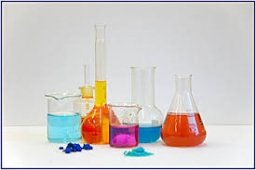Did you find an error or inaccuracy? Feel free to write us. Thank you!Tips to related online calculators
Looking for a statistical calculator?
Tip: Our volume units converter will help you with the conversion of volume units.

## Related math problems and questions:

• Water tanksA different amount of water is poured into each of the three identical vessels. In the first vessel, water fills 30% of its volume and in the second vessel 40% of its volume. The third container contains 19 liters of water. If we distributed the water fro
• PeroxideHow many ml should we pour 30% of peroxide (H2O2) into 100ml H2O to give a 20% solution?
• A 36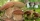A 36 g sample of the substance contains 91% water. After drying, the weight of the sample was reduced to 18 g. What percentage of water is now in the sample?
• SolutionsHow much 60% solution and how much 35% solution is needed to create 100 l of 40% solution?
• Clogging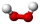How much-distilled water must the pharmacist add to 30g of a 30% hydrogen peroxide solution to obtain a 3% solution to clogging?
• Solutions, mixturesHow many liters of 70% solution we must add to 5 liters of 30% solution to give us a 60% solution?
• Final examThere are 5 learners in a class that has written a final exam Aleta scored 55% vera scored 36% and Sibusiso scored 88% if Thoko scored 71% and the class average was 63%. What was Davids score as a percentage?
• Hydrochloric acidDetermine the concentration of which must have a solution of hydrochloric acid that mixing 10 l of the solution with 8 liters of 26% solution to get the solution with a concentration of 50%?
• Solutions, mixturesWe have 2 liters of 20% solution available. How much 70% solution do we need to add to it to get a 30% solution?
• Alcohol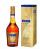How many cubic centimeters of 96% alcohol is needed to obtain 0.75 liters of 40% alcohol?
• Vinegar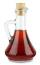What percentage vinegar we get if we mix 1 if dm³ eight percent vinegar with 1.5 dm³ six percent vinegar?
• Mixture 2How many liters of water must be added to 7 liters of a 20% solution to obtain a 10% solution?
• PharmacyThe pharmacy adds to 3 liters of 95-percent alcohol 5 liters of 38.04 percent alcohol. How much percent alcohol the pharmacy got?
• ThereThere is 5 l of water with a temperature of 20°C in the bucket. We add 3 liters of hot water. The resulting temperature will be 30°C. What was the temperature of the hot water?
• Alcohol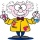6l 48% alcohol, how much 52% alcohol must be added to give 50% alcohol?
• Alcohol mixing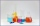How much 64% of the alcohol must be poured into 6 liters of 85% alcohol to produce 79% alcohol?
• Kerosine and petrol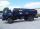If 4 liters of petrol containing 15% kerosine are added to another 7 liters of petrol containing 10% kerosine, what percentage of the petrol is kerosine?